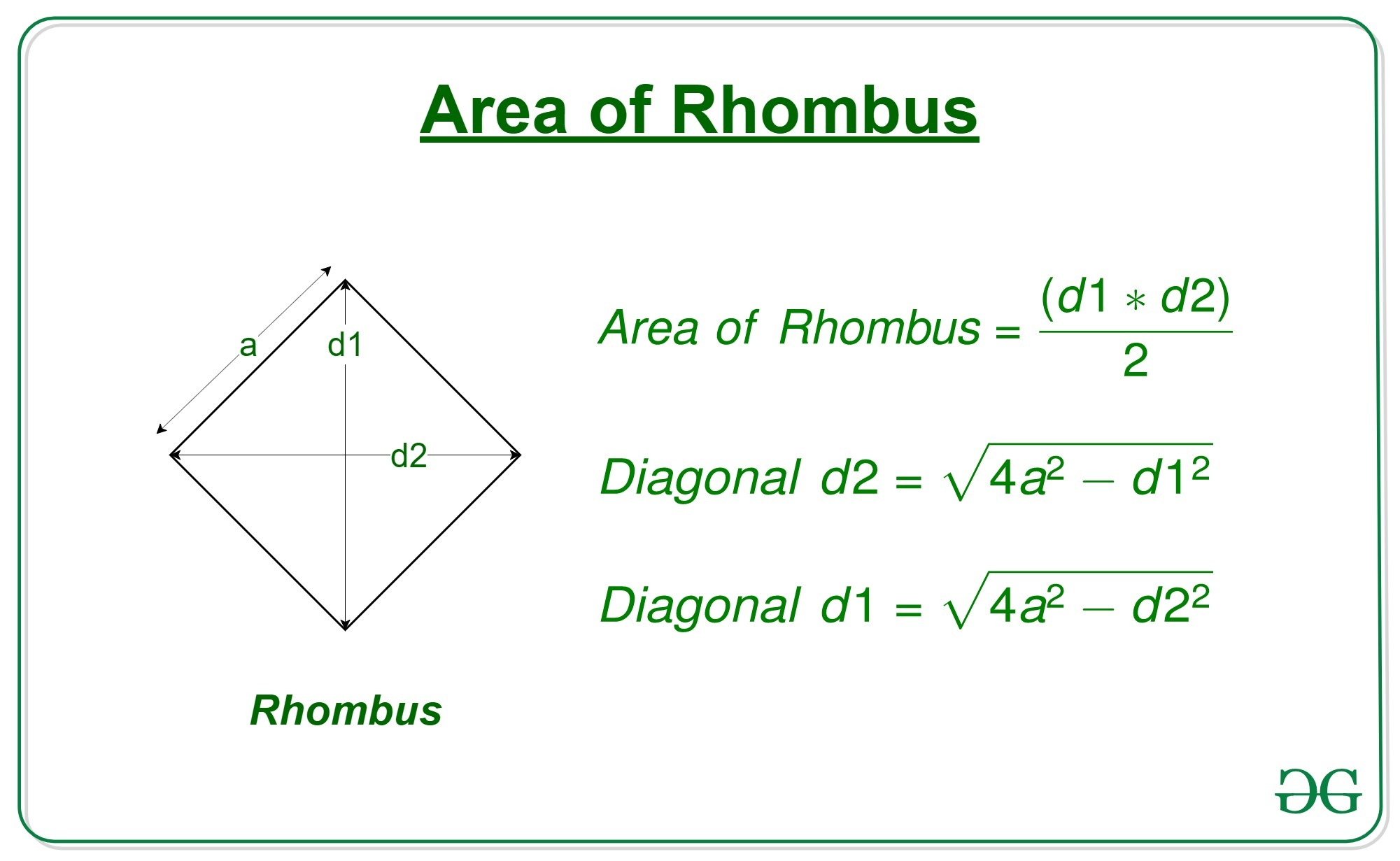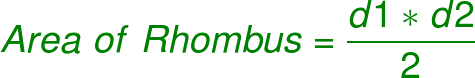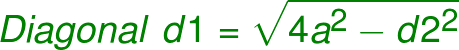GFG App
Open AppBrowser
Continue

# Program to calculate area of a rhombus whose one side and diagonal are given

Given the length of diagonal ‘d1’ of a rhombus and a side ‘a’, the task is to find the area of that rhombus.

A rhombus is a polygon having 4 equal sides in which both the opposite sides are parallel, and opposite angles are equal.Examples:

```Input: d = 15, a = 10
Output: 99.21567416492215

Input: d = 20, a = 18
Output: 299.3325909419153```

Approach:

• Get the diagonal ‘d1’ and side ‘a’ of the rhombus
• We know that,•
• But since we don’t know the other diagonal d2, we cannot use this formula yet
• So we first find the second diagonal d2 with the help of d1 and a•
• Now we can use the area formula to compute the area of the Rhombus

## C++

 `// C++ program to calculate the area of a rhombus` `// whose one side and one diagonal is given` `#include` `using` `namespace` `std;`   `// function to calculate the area of the rhombus` `double` `area(``double` `d1, ``double` `a)` `{` `    `  `    ``// Second diagonal` `    ``double` `d2 = ``sqrt``(4 * (a * a) - d1 * d1);`   `    ``// area of rhombus` `    ``double` `area = 0.5 * d1 * d2;`   `    ``// return the area` `    ``return` `area;` `}`   `// Driver code` `int` `main()` `{` `    ``double` `d = 7.07;` `    ``double` `a = 5;` `    ``printf``(``"%0.8f"``, area(d, a));` `}`   `// This code is contributed by Mohit Kumar`

## Java

 `// Java program to calculate the area of a rhombus` `// whose one side and one diagonal is given` `class` `GFG` `{`   `    ``// function to calculate the area of the rhombus` `    ``static` `double` `area(``double` `d1, ``double` `a)` `    ``{` `        `  `        ``// Second diagonal` `        ``double` `d2 = Math.sqrt(``4` `* (a * a) - d1 * d1);` `    `  `        ``// area of rhombus` `        ``double` `area = ``0.5` `* d1 * d2;` `    `  `        ``// return the area` `        ``return` `area;` `    ``}` `    `  `    ``// Driver code` `    ``public` `static` `void` `main (String[] args) ` `    ``{` `        ``double` `d = ``7.07``;` `        ``double` `a = ``5``;` `        ``System.out.println(area(d, a));` `    ``}` `}`   `// This code is contributed by AnkitRai01`

## Python3

 `# Python program to calculate` `# the area of a rhombus` `# whose one side and ` `# one diagonal is given`   `# function to calculate ` `# the area of the rhombus` `def` `area(d1, a):` `    `  `    ``# Second diagonal` `    ``d2 ``=` `(``4``*``(a``*``*``2``) ``-` `d1``*``*``2``)``*``*``0.5` `    `  `    ``# area of rhombus` `    ``area ``=` `0.5` `*` `d1 ``*` `d2` `    `  `    ``# return the area` `    ``return``(area)`   `# driver code` `d ``=` `7.07` `a ``=` `5` `print``(area(d, a))`

## C#

 `// C# program to calculate the area of a rhombus` `// whose one side and one diagonal is given` `using` `System;`   `class` `GFG` `{`   `    ``// function to calculate the area of the rhombus` `    ``static` `double` `area(``double` `d1, ``double` `a)` `    ``{` `        `  `        ``// Second diagonal` `        ``double` `d2 = Math.Sqrt(4 * (a * a) - d1 * d1);` `    `  `        ``// area of rhombus` `        ``double` `area = 0.5 * d1 * d2;` `    `  `        ``// return the area` `        ``return` `area;` `    ``}` `    `  `    ``// Driver code` `    ``public` `static` `void` `Main (String []args) ` `    ``{` `        ``double` `d = 7.07;` `        ``double` `a = 5;` `        ``Console.WriteLine(area(d, a));` `    ``}` `}`   `// This code is contributed by Arnab Kundu`

## Javascript

 ``

Output:

`24.999998859949972`

Time Complexity: O(log(n)) as inbuilt sqrt function is used

Auxiliary Space: O(1)

My Personal Notes arrow_drop_up